# Ideal Gas Law Formulas

Ideal Gas Law Formulas

In an ideal gas, there are no attractive forces between the gas molecules. This is a good approximation for most gases. An ideal gas has three variables that define its state. They are: absolute pressure (P), volume (V), and absolute temperature (T). The ideal gas law defines how these state variables relate to each other. There are two forms, one defined in terms of the number of moles (mol) of gas, and one defined in terms of the number of molecules of gas. One mole of a substance consists ofmolecules. Pressure is measured in Pascals (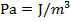), volume is measured in cubic meters (m3), and temperature is measured in Kelvin (K).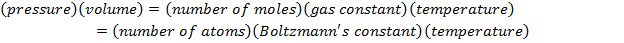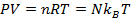P = pressure (Pa)

V = volume (m3)

n = number of moles of gas (mol)

R = gas constant ()

T = temperature (K)

N = number of molecules of gas (unitless)

kB = Boltzmann's constant (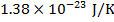)

Ideal Gas Law Formulas Questions:

1) Standard Temperature and Pressure (STP) is defined to beand(kilo-Pascals). What is the volume of one mole (1 mol) of an ideal gas at STP? Give your answer in liters (L) where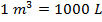.

Answer: The volume of the gas can be found by rearranging the molar form of the ideal gas formula: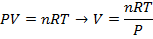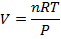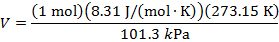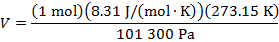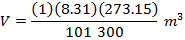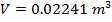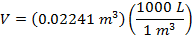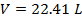The volume of one mole of an ideal gas at standard temperature and pressure is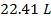.

2) If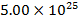molecules of an ideal gas are compressed in a volume of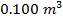at a temperature of, what is the pressure of the gas?

Answer: The pressure of the gas can be found by rearranging the molecular form of the ideal gas formula: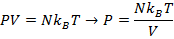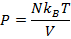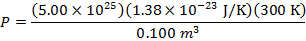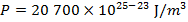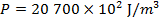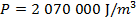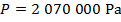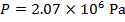The pressure ofmolecules of an ideal gas compressed in a volume ofat a temperature ofis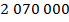Pascals, which can also be written as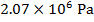, or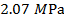(mega-Pascals).